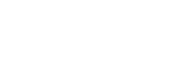## Questions

1. State two differences between the cathode ray tube (CRT) of a T.V and the cathode ray oscilloscope (CRO)
2. Distinguish between a photon and a quantum.
3. How does the energy of ultra violet light compare to that of yellow light given that the energy E of a wave frequency f, is given by E = hf, where h is plank’s constant?
4. A photon has an energy of 5x10-19J. Calculate the wavelength associated with this photon.
5. The control grid in a cathode Ray Oscilloscope (CRO) is used to control brightness of the beam on the screen. How is this achieved?
6.
1. The figure below shows the features of a cathode ray tube.1. Name the parts labelled A and B. (2mks)
2. Explain how the electrons are produced in tube. (2mks)
3. State two functions of the anodes. (2mks)
4. At what part of the cathode ray tube would the time base be connected? (1mk)
5. Why is a vacuum created in the tube? (1mk)
2. The graph in the figure below was obtained on a cathode ray oscilloscope (CRO) screen when the output of an a.c generator was connected to the input of the CRO. The time-base calibration of the CRP was set at 20 milliseconds per centimeter and the y- gain at 5 volts per centimeter.1. Determine the pick voltage of the generator. (2mks)
2. Determine the frequency of the voltage. (3mks)
3. On the same grid, redraw the graph for the same voltage when the time base calibration is set at 40 milliseconds per centimeter and the 7-gain at 10 volts per centimeter. (Show at least one complete cycle). (2mks)
7. The control grid in a cathode ray oscilloscope (CRO) is used to control the brightness of the beam on the screen. Explain how this is achieved.
8. State and explain three uses of main parts of a CRT in an oscilloscope.
9. Sketch the picture seen on the screen of a cathode ray oscilloscope when the oscilloscope is adjusted so that the spot is in the middle of the screen and the output terminals from a transformer connected to the mains are connected across the Y-plates.
10. The diagram shows the screen of a cathode ray tube, and behind it the position of the X and y plates which deflect the electron beam. The beam forms a spot on the screen.1. Draw a labelled diagram showing a side view of the cathode ray tube.
2. How is the brightness of the spot controlled?
3. The “X-shift” control on the front of the cathode ray oscilloscope moves the spot sideways on the screen. What kind of voltage direct, alternating or zero) does it apply to:
1. The X plates
2. The Y plates
11. The ‘time–base’ voltage normally applied to the X-plates in a RCO varies with time as shown.1. Describe the motion of the spot when the time-base is on.
2. Illustrate on the diagram above what is seen on the screen if an alternating voltage is applied to the Y-plates with the time-base on.
3. State two uses of the CRO.

1. CRT
- Produces 625 times per second
- Has two time bases
- forms an image.
- Deflection is caused by magnetic field.
- displays pictures.

CRO
- Produces 25 lines per second
- Has one time base
- displays waves
- forms a spot on screen
- Deflection is caused by electric field.
2. Photon – particle of light energy.
Quantum – Packet of energy.
3. Ultra violet has a higher energy than yellow light.
4. E = hf = hc
λ
λ = 6.63 x 10-34 x 3 x 108
5 x 10-19
λ = 3.978 x 10-7
5. Low negative voltage is applied on control grid, which controls the number of electrons reaching the screen.
6.
1.
1. A – Grid
B – Filament
2. Filament heats cathode electron boil off cathode (theremionic emission)
3. - Accelerating
- Focusing.
4. Across X-plates.
5. to reduce collision with air molecules that could lead to ionization.
2.
1. Height = 4cm
Peak value = 4 x 5 = 20V
2. 2 wavelength = 16cm
T = 8 x 20 x 10-3
= 0.16s
F = 1/T = 1/0.16 = 6.25Hz
3.7. Low negative voltage is applied on control grid, which control the number of electrons reaching the screen.
8.
1. Electron gun – produces direct electrons.
2. Deflecting system – deflecting the beam to necessitate the study of external circuit.
3. Fluorescent screen – to display the pattern being studied.

#### Download Cathode Rays and Cathode Ray Tube Questions and Answers - Physics Form 4 Topical Revision.

• ✔ To read offline at any time.
• ✔ To Print at your convenience
• ✔ Share Easily with Friends / Students